877-601-6284
Get Started by Finding a Local Center

# What is Infinity?

Jun 13, 2017 | Location Pflugerville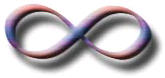Infinity ... ... it's not big ... ... it's not huge ... ... it's not tremendously large ... ... it's not extremely humongously enormous ... ... it's ...

Endless!

### Infinity has no end

Infinity is the idea of something that has no end.

In our world we don't have anything like it. So we imagine traveling on and on, trying hard to get there, but that is not actually infinity.

So don't think like that (it just hurts your brain!). Just think "endless", or "boundless".

If there is no reason something should stop, then it is infinite.

### Infinity does not grow

Infinity is not "getting larger", it is already fully formed.

Sometimes people (including me) say it "goes on and on" which sounds like it is growing somehow. But infinity does not do anything, it just is.

### Infinity is not a real number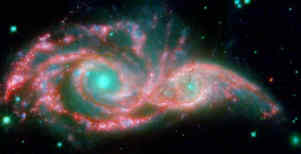Infinity is not a real number, it is an idea. An idea of something without an end.

Infinity cannot be measured.

Even these faraway galaxies can't compete with infinity.

### Infinity is Simple

Yes! It is actually simpler than things which do have an end. Because when something has an end, we have to define where that end is.### Example: in Geometry a Line has infinite length.

A Line goes in both directions without end.

When there is one end it is called a Ray, and when there are two ends it is called a Line Segment, but they need extra information to define where the ends are.

So a Line is actually simpler then a Ray or Line Segment.

### More Examples:

{1, 2, 3, ...}

The sequence of natural numbers never ends, and is infinite.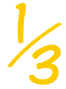OK, 1/3 is a finite number (it is not infinite). But written as a decimal number the digit 3 repeats forever (we say "0.3 repeating"):

0.3333333... (etc)

There's no reason why the 3s should ever stop: they repeat infinitely.

0.999...

So, when we see a number like "0.999..." (i.e. a decimal number with an infinite series of 9s), there is no end to the number of 9s.

You cannot say "but what happens if it ends in an 8?", because it simply does not end. (This is why 0.999... equals 1).

AAAA...

An infinite series of "A"s followed by a "B" will NEVER have a "B".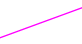There are infinite points in a line. Even a short line segment has infinite points.

## Big Numbers

There are some really impressively big numbers.

Googol is 1 followed by one hundred zeros (10100) :

10,000,000,000,000,000,000,000,000,000,000,000,
000,000,000,000,000,000,000,000,000,000,000,
000,000,000,000,000,000,000,000,000,000,000

A Googol is already bigger than the number of elementary particles in the known Universe, but then there is the Googolplex. It is 1 followed by Googol zeros. I can't even write down the number, because there is not enough matter in the known universe to form all the zeros:

10,000,000,000,000,000,000,000,000,000,000,000,000, ... (Googol number of Zeros)

And there are even larger numbers that need to use "Power Towers" to write them down.

For example, a Googolplex can be written as this power tower: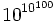That is ten to the power of (10 to the power of 100),

But imagine an even bigger number like(which is a Googolplexian).

And we can easily create much larger numbers than those!

### Finite

All of these numbers are "finite", we could eventually "get there".

But none of these numbers are even close to infinity. Because they are finite, and infinity is ... not finite!

## Using Infinity

We can sometimes use infinity like it is a number, but infinity does not behave like a real number.

To help you understand, think "endless" whenever you see the infinity symbol "":

### Example: ∞ + 1 = ∞

Which says that infinity plus one is still equal to infinity.

When something is already endless, we can add 1 and it is still endless.

The most important thing about infinity is that:

 -∞ < x < ∞ Where x is a real number

Which is mathematical shorthand for
"negative infinity is less than any real number,
and infinity is greater than any real number"

Here are some more properties:

Special Properties of Infinity
+  =
- + - = -

×  =
- × - =
- ×  = -

x +  =
x + (-) = -
x -  = -
x - (-) =

For x>0 :
x ×  =
x × (-) = -∞
For x<0 :
x ×  = -∞
x × (-) =

## Undefined Operations

All of these are "undefined":

"Undefined" Operations
0 ×
0 × -
+ -
-
/
0
1

### Example: Isn't ∞ / ∞ equal to 1?

No, because we really don't know how big infinity is, so we can't say that two infinities are the same. For example  +  = , so

 ∞∞ = ∞ + ∞∞ which  means that: 11 = 21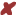And that doesn't make sense!

I could have also made 1=3 and so on ... so we say that  /  is undefined.

## Infinite Sets

If you continue to study this subject you will find discussions about infinite sets, and the idea of different sizes of infinity.

That subject has special names like Aleph-null (how many Natural Numbers), Aleph-one and so on, which are used to measure the sizes of sets.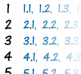For example, there are infinitely many whole numbers {0,1,2,3,4,...},

But there are more real numbers (such as 12.308 or 1.1111115) because there are infinitely many possible variations after the decimal place as well.

But that is an advanced topic, and goes beyond the simple concept of infinity we discuss here.

## Conclusion

Infinity is a simple idea: "endless". Most things we know have an end, but infinity does not.

The original article from mathisfun.com can be found HERE

##### Locations near
ME
Capitol Hill DC 28.48 mi
621 Pennsylvania Ave SE
1st-floor unit
Washington, DC 20003